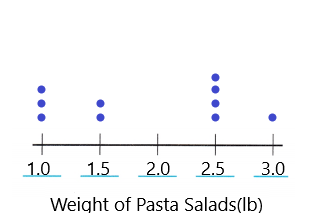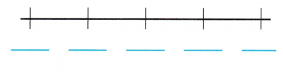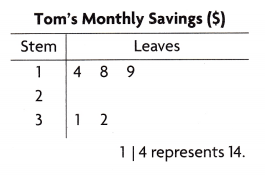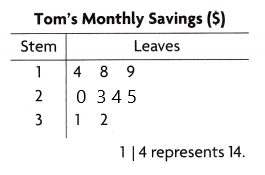Refer to our Texas Go Math Grade 5 Answer Key Pdf to score good marks in the exams. Test yourself by practicing the problems from Texas Go Math Grade 5 Module 16 Assessment Answer Key.

Vocabulary

Choose the best term from the box.

Question 1.
A ___ is a graph that shows frequency of data along a number line. (p. 571)
A line plot is a graph that shows the frequency of data along a number line.

Question 2.
A _______ is a graph that shows a relationship between two sets of data. (p. 595)
A scatter plot is a graph that shows a relationship between two sets of data.

Concepts and Skills

Use the data and the dot plot for 3-4.Question 3.
Complete a dot plot of the data. TEKS 5.9.
The dot plot of the data isQuestion 4.
What is the weight of the greatest number of pasta salads? TEKS 5.9.3.0 lb.

Explanation:
The weight of the greatest number of pasta salads is 3.0 lb.

Use the table and the scatter plot for 5-7.Question 5.
Complete a scatter plot of the data. TEKS 5.9.B

Question 6.
As the number of days traveled increases, does the distance traveled increase, decrease, or stay about the same? TEKS 5.9.CQuestion 7.
About how many miles would you predict Sally would travel in 6 days? TEKS 5.9.C

Texas Test Prep

The amount of money Tom saved each month in dollars is 23, 18, 24, 32, 27, 31, 25, 20,14, and 19. Use the data and the stem-and-leaf plot for 8-10.

Question 8.
Tom is making a stem-and-leaf plot of the data. What are the leaves for stem 2? TEKS 5.9.A
(A) 3, 4, 5, 7
(B) 0, 3, 4, 5
(C) 0, 3, 3, 5, 7
(D) 0, 3, 4, 5, 7B.

Explanation:
The leaves for stem 2 are 0, 3, 4, 5.Question 9.
For how many months was Tom’s savings less than $20? TEKS 5.9C (A) 2 months (B) 5 months (C) 3 months (D) 4 months Answer: C. Explanation: There are 3 months that Tom’s savings are less than$20.

Question 10.
Tom plans to save twice as much next month as he did during the months he saved over $30 a month. How much will Tom save next month? TEKS 5.9.C (A)$63
(B) $126 (C)$86
(D) $43 Answer: A. Explanation: Tom saves 31+32 =$63 for next month.

Kim bought scraps of ribbon at a discount store. The length of each ribbon in inches is 9, 8$$\frac{3}{4}$$, 8$$\frac{1}{2}$$, 8$$\frac{3}{4}$$, 8$$\frac{1}{4}$$, 8$$\frac{1}{4}$$, 8$$\frac{3}{4}$$, 8$$\frac{1}{2}$$, 8$$\frac{3}{4}$$, 8$$\frac{1}{4}$$ and 8. Use the data and the dot plot for 11-12.

Question 11.
Kim is completing the dot plot of the data. How many dots should she put above 8$$\frac{3}{4}$$ ? TEK 5.9.C
(A) 1
(B) 4
(C) 3
(D) 24.

Explanation:
The number of dots did she put above 8$$\frac{3}{4}$$ is 4.Question 12.
What is the range of ribbon lengths în inches? TEKS 5.9.C.
Record your answer and fill in the bubbles on the grid. Be sure to use the correct place value.